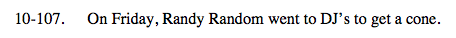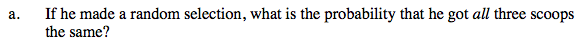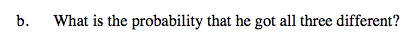### Home > A2C > Chapter Ch10 > Lesson 10.2.4 > Problem10-107

10-107.Once the first scoop is chosen, there is no choice for the second or third scoop.

$\frac{5}{1^{\text{st}}}\cdot \frac{1}{2^{\text{nd}}}\cdot \frac{1}{3^{\text{rd}}}=5\text{ ways}$

$P\text{(all the same)}=\frac{\text{number of ways all the same}}{\text{total number of ways}}=\frac{5}{5\cdot5\cdot5}$

$\frac{5}{125}=0.04$Since the order does matter, it is the number of ways 3 out of 5 scoops can be arranged.

$\frac{5\cdot4\cdot3}{125}$